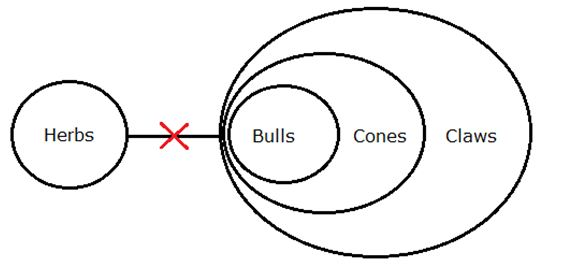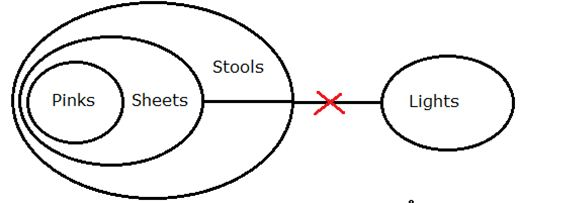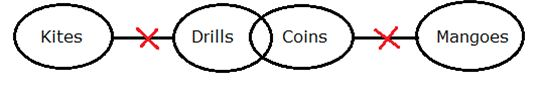# IBPS Clerk Prelims Reasoning Ability Questions 2019 (Day-21)

Dear Aspirants, Our IBPS Guide team is providing new series of Reasoning Questions for IBPS Clerk Prelims 2019 so the aspirants can practice it on a daily basis. These questions are framed by our skilled experts after understanding your needs thoroughly. Aspirants can practice these new series questions daily to familiarize with the exact exam pattern and make your preparation effective.

[WpProQuiz 7460]

Ensure Your Ability Before of Exam – Take IBPS Clerk Prelims Free Mock test

Inequality

Directions (1-4): In all the questions that follow, relationship between different elements is shown in the statements. The statements are followed by two conclusions.

a) Only conclusion I follows

b) Only conclusion II follows

c) Either conclusion I or conclusion II follows

d) Neither conclusion I nor conclusion II follows

e) Both conclusion I and conclusion II follows

1) Statements:

A > B ≤ F = C, F ≤ P ≤ T, C ≤ D

Conclusions:

I. T > D

II. D ≥ T

2) Statements:

P > Q ≥ R, T = R ≤ A, A ≥ B

Conclusions:

I. B < P

II. P ≤ B

3) Statements:

T > A < B ≤ C, Q ≤ L ≤ A, G < L

Conclusions:

I. C > G

II. Q < T

4) Statements:

P > Q, Q < M, M ≥ A

Conclusions:

I. A < Q

II. P > M

Misc

5) If all the letters of the word “PRODUCTIVE” are arranged as per the English alphabetical order starting from the left end, then the position of how many letters will remain unchanged?

a) More than three

b) Three

c) None

d) One

e) Two

6) What comes in the place of Question mark in the given series?

‘LMNOPQRKLMNOPJKLMN?’

a) O

b) I

c) P

d) H

e) J

7) How many such pairs of letters are there in the word “WONDERFUL” each of which has as many letters between them in the word as in the English alphabet (both backward and forward direction)?

a) 1

b) 2

c) 3

d) 4

e) More than 4

Syllogism

Direction (8-10): In each of the questions below are given some statements followed by two conclusions numbered I and II. You have to take the given statements to be true even if they seem to be at variance from commonly known facts. Read all the conclusions and then decide which of the given conclusions logically follows from the given statements disregarding commonly known facts.

8) Statements:

All cones are claws.

No claws is a herbs.

All bulls are cones.

Conclusions:

I. Some herbs are cones.

II. No bull is a herbs.

a) If only Conclusion I follows.

b) If only Conclusion II follows.

c) If either Conclusion I or II follows.

d) If neither Conclusion I nor II follows.

e) If both Conclusions I and II follow.

9) Statements:

All pinks are sheets.

All sheets are stools.

No sheet is a light.

Conclusions:

I. No light is a stool.

II. Some stools are pinks.

a) If only Conclusion I follows.

b) If only Conclusion II follows.

c) If either Conclusion I or II follows.

d) If neither Conclusion I nor II follows.

e) If both Conclusions I and II follow.

10) Statements:

Some drills are coins.

No coins are mangoes.

No drills are kites.

Conclusions:

I. Some kites are coins.

II. Some mangoes are drills.

a) If only Conclusion I follows.

b) If only Conclusion II follows.

c) If either Conclusion I or II follows.

d) If neither Conclusion I nor II follows.

e) If both Conclusions I and II follow.

Directions (1-4):

T ≥ F = C ≤ D, this gives both T and D less than equal to F

So either T will be > D or T will be ≤ D

B ≤ A ≥R < P. It satisfies the condition of either or case;

so, either conclusion I or conclusion II follows.

C > A ≥ L > G, so C > G

Q ≤ L ≤ A < T, so Q < T

A ≤ M > Q, so no relationship between A and Q

P > Q < M, both P and M less than Q so no relationship between P and M

If all the letters of the word “PRODUCTIVE” are arranged in English alphabetical, then it becomes-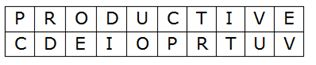Thus, we see,

None of the letters remain at the same position.

The given series can be written as follows: LMNOPQR / KLMNOP / JKLMN / ?

thus, according to the English alphabetical series, two letters are removed in each step and one letter is added at the first place in each step.

For ex: after LMNOPQR – K is added before L, according to alphabetical series and, QR are removed. Same is followed for next steps and, thus, at the place of ?

we get, “I” as next group of letters would be – IJKL

4 such pairs of letter are there in the word “WONDERFUL” each of which letters between them in the word as in the alphabet.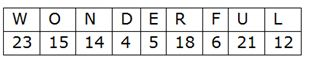WR, ON, OU, DE re the four pairs

Direction (8-10):